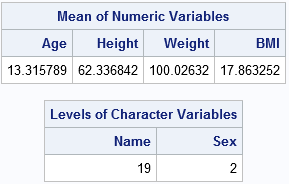Prior to SAS/IML 14.2, every variable in the Interactive Matrix Language (IML) represented a matrix. That changed when SAS/IML 14.2 introduced two new data structures: data tables and lists. This article gives an overview of data tables. I will blog about lists in a separate article.

A matrix is a rectangular array that contains all numerical or all character values. Numerical matrices are incredibly useful for computations because linear algebra provides a powerful set of tools for implementing analytical algorithms. However, a matrix is somewhat limiting as a data structure. Matrices are two-dimensional, rectangular, and cannot contain mixed-type data (numeric AND character). Consequently, you can't use one single matrix to pass numeric and character data to a function.

Data tables in SAS/IML are in-memory versions of a data set. They contain columns that can be numeric or character, as well as column attributes such as names, formats, and labels. The data table is associated with a single symbol and can be passed to modules or returned from a module. the TableCreateFromDataSet function, as shown:

```proc iml; tClass = TableCreateFromDataSet("Sashelp", "Class"); /* SAS/IML 14.2 */```

The function reads the data from the Sashelp.Class data set and creates an in-memory copy. You can use the tClass symbol to access properties of the table. For example, if you want to obtain the names of the columns in the table, you can use

 ```varNames = TableGetVarName(tClass); print varNames;```### Extracting columns and adding new columns

```Y = TableGetVarData(tClass, {"Weight" "Height"}); wt = Y[,1]; ht = Y[,2]; /* get Height and Weight variables */ BMI = wt / ht##2 * 703; /* BMI formula */ call TableAddVar(tClass, "BMI", BMI); /* add new "BMI" column to table */```

### Passing data tables to modules

As indicated earlier, you can use data tables to pass mixed-type data into a user-defined function. For example, the following statements define a module whose argument is a data table. The module prints the mean value of the numeric columns in the table, and it prints the number of unique levels for character columns. To do so, it first extracts the numeric data into a matrix, then later extracts the character data into a matrix.

```start QuickSummary(tbl); type = TableIsVarNumeric(tbl); /* 0/1 vector */ /* for numeric columns, print mean */ idx = loc(type=1); /* numeric cols */ if ncol(idx)>0 then do; /* there is a numeric col */ varNames = TableGetVarName(tbl, idx); /* get names */ m = TableGetVarData(tbl, idx); /* extract numeric data */ mean = mean(m); print mean[colname=varNames L="Mean of Numeric Variables"]; end; /* for character columns, print number of levels */ idx = loc(type=0); /* character cols */ if ncol(idx)>0 then do; /* there is a character col */ varNames = TableGetVarName(tbl, idx); /* get names */ m = TableGetVarData(tbl, idx); /* extract character data */ levels = countunique(m, "col"); print levels[colname=varNames L="Levels of Character Variables"]; end; finish;   run QuickSummary(tClass);```### Summary

SAS/IML 14.2 supports data tables, which are rectangular arrays of mixed-type data. You can use built-in functions to extract columns from a table, add columns to a table, and query the table for attributes of columns. For more information about data tables, see the chapter

The post Data tables: Nonmatrix data structures in SAS/IML appeared first on The DO Loop.